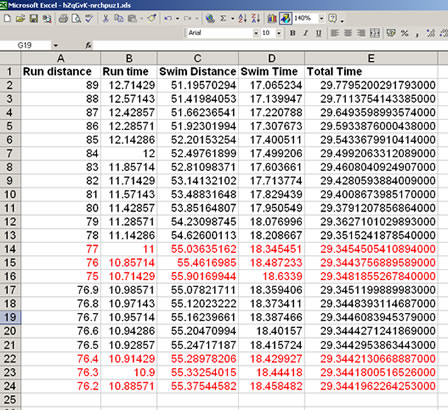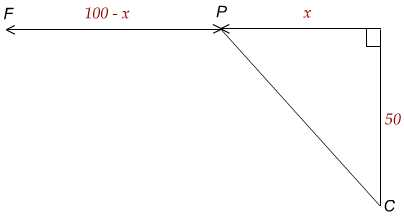#### You may also like### Fixing the Odds

You have two bags, four red balls and four white balls. You must put all the balls in the bags although you are allowed to have one bag empty. How should you distribute the balls between the two bags so as to make the probability of choosing a red ball as small as possible and what will the probability be in that case?### Scratch Cards

To win on a scratch card you have to uncover three numbers that add up to more than fifteen. What is the probability of winning a prize?### Escalator

At Holborn underground station there is a very long escalator. Two people are in a hurry and so climb the escalator as it is moving upwards, thus adding their speed to that of the moving steps. ... How many steps are there on the escalator?

# Where to Land

##### Age 14 to 16 Challenge Level:

We had a number of correct answers to this problem. Several solutions used a spreadsheet, for example the one shown below is from an anonymous solver. We also received a solution from someone who has done some calculus and was able to solve the problem using this approach. I have included this solution at the end for those of you who are interested.

My disappointment is that some of you are still just sending in answers without explanation and I do want to see how you arrived at your answers. Although the solution below was found using a spreadsheet you might have used a calculator and carried out a standard "trial and improvement" process to obtain the same result.

Catherine, Ellean, Anna from The Mount School, York add the following comment which means they were really thinking about the numbers and the context...

"P.S she must be super woman to run and swim that fast."

How fast do you think you could run and swim in m/s?

Andisheh of Springfield School offered the following explanation (well done):

First of all I tried using trial and error. I thought the more she runs the quicker she would get thre but that did not work. I used Pythagoras to work out the distances swum and run. I tried drawing a table but that did not help a lot. Idrew a graph and trid to find out the lowest point but I was a bit inaccurate. Then I used Microsoft Excel and typed in the formula and tried it with numbers from 0 to 100 and tried to find our the shortest time and this worked.

Andisheh attached a spreadsheet and I have used this and several other solutions employing a spreadhsheet to create the extract below: Solvers were able to find that the minimum time occurred with a distance between 76 and 77m and then worked with one and then two decimal places to obtain greater accuruacy.

"Swim Distance" was calculated using Pythagoras' theorem:
The right-angled triangle will have sides of 50m (the distance from the beach) and $x$m, which is 100m (the distance along the beach) minus the distance run.

$$\mbox{Swim}^2 = 50^2 + x^2$$

$$\mbox{Distance swum} = \sqrt{50^2 + x^2}$$

The times were then found in seconds by dividing the distances by the speeds and the total time just required the two times to be added.The final solution was sent in by an anonymous solver who used calculus (a branch of mathematics normally met for the first time at advanced level in the UK). It is a neat solution but this high level mathematics was certainly not required!Let $T$ be the total time.

Let $t_1$ be the time spent swimming.

Let $t_2$ be the time spent running.

By Pythagoras' theorem

\begin{eqnarray} PC^2 &=& 50^2 + x^2 \\ PC&=& \sqrt{50^2 + x^2} \end{eqnarray}

So for the total time:

$$T = \frac{\sqrt{x^2 + 50^2}}{3} + \frac{100-x}{7}$$

$$\frac{dT}{dx} = \frac{x(x^2 + 50^2)^\frac{-1}{2}}{3}- \frac{1}{7}$$

$$\frac{dT}{dx} = 0 \mbox{ when } \frac{1}{7} = \frac{x(x^2 + 50^2)^\frac{-1}{2}}{3}$$ Important question to think about:

How would you check that this is a minimum value?

Solving the equation above for $x$

\begin{eqnarray} 3(x^2+50^2)^\frac{1}{2} &=& 7x\\ 9(x^2+50^2)&=& 49x^2\\ x&=& 23.7 \mbox{ (1dp)} \end{eqnarray}

Chris will need to land at the point on the beach 76.3m from the family.